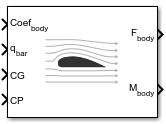Documentation

### This is machine translation

Mouseover text to see original. Click the button below to return to the English version of the page.

Note: This page has been translated by MathWorks. Click here to see
To view all translated materials including this page, select Country from the country navigator on the bottom of this page.

# Aerodynamic Forces and Moments

Compute aerodynamic forces and moments using aerodynamic coefficients, dynamic pressure, center of gravity, center of pressure, and velocity

## Library

Aerodynamics## Description

The Aerodynamic Forces and Moments block computes the aerodynamic forces and moments about the center of gravity. By default, the inputs and outputs are represented in the body axes.

Let α be the angle of attack and β the sideslip. The rotation from body to stability axes:

`${C}_{s←b}=\left[\begin{array}{ccc}\mathrm{cos}\left(\alpha \right)& 0& \mathrm{sin}\left(\alpha \right)\\ 0& 1& 0\\ -\mathrm{sin}\left(\alpha \right)& 0& \mathrm{cos}\left(\alpha \right)\end{array}\right]$`

can be combined with the rotation from stability to wind axes:

`${C}_{w←s}=\left[\begin{array}{ccc}\mathrm{cos}\left(\beta \right)& \mathrm{sin}\left(\beta \right)& 0\\ -\mathrm{sin}\left(\beta \right)& \mathrm{cos}\left(\beta \right)& 0\\ 0& 0& 1\end{array}\right]$`

to yield the net rotation from body to wind axes:

`${C}_{w←b}=\left[\begin{array}{ccc}\mathrm{cos}\left(\alpha \right)\mathrm{cos}\left(\beta \right)& \mathrm{sin}\left(\beta \right)& \mathrm{sin}\left(\alpha \right)\mathrm{cos}\left(\beta \right)\\ -\mathrm{cos}\left(\alpha \right)\mathrm{sin}\left(\beta \right)& \mathrm{cos}\left(\beta \right)& -\mathrm{sin}\left(\alpha \right)\mathrm{sin}\left(\beta \right)\\ -\mathrm{sin}\left(\alpha \right)& 0& \mathrm{cos}\left(\alpha \right)\end{array}\right]$`

Moment coefficients have the same notation in all systems. Force coefficients are given below. Note there are no specific symbols for stability-axes force components. However, the stability axes have two components that are unchanged from the other axes.

`${F}_{A}^{w}\equiv \left[\begin{array}{c}-D\\ -C\\ -L\end{array}\right]={C}_{w←b}\cdot \left[\begin{array}{c}{X}_{A}\\ {Y}_{A}\\ {Z}_{A}\end{array}\right]\equiv {C}_{w←b}\cdot {F}_{A}^{b}$`
Components/Axesxyz
WindCDCCCL
StabilityCYCL
BodyCXCYCZ (–CN)

Given these definitions, to account for the standard definitions of D, C, Y (where Y = -C), and L, force coefficients in the wind axes are multiplied by the negative identity diag(-1, -1, -1). Forces coefficients in the stability axes are multiplied by diag(-1, 1, -1). CN and CX are, respectively, the normal and axial force coefficients (CN = -CZ).

## Parameters

Input Axes

Specifies coordinate system for input coefficients: `Body` (default), `Stability`, or `Wind`.

Force Axes

Specifies coordinate system for aerodynamic force: `Body` (default), `Stability`, or `Wind`.

Moment Axes

Specifies coordinate system for aerodynamic moment: `Body` (default), `Stability`, or `Wind`.

Reference area

Specifies the reference area for calculating aerodynamic forces and moments.

Reference span

Specifies the reference span for calculating aerodynamic moments in x-axes and z-axes.

Reference length

Specifies the reference length for calculating aerodynamic moment in the y-axes.

## Inputs and Outputs

The first input consists of aerodynamic coefficients (in the chosen input axes) for forces and moments. These coefficients are ordered into a vector depending on the choice of axes:

Input AxesInput Vector
Body(axial force Cx, side force Cy, normal force Cz, rolling moment Cl, pitching moment Cm, yawing moment Cn)
Stability(drag force CD(β=0), side force Cy, lift force CL, rolling moment Cl, pitching moment Cm, yawing moment Cn)
Wind(drag force CD, cross-wind force Cc, lift force CL, rolling moment Cl, pitching moment Cm, yawing moment Cn)
InputDimension TypeDescription
Second Contains the dynamic pressure.
Third Contains the center of gravity.
Fourth Contains the center of pressure. This can also be taken as any general moment reference point as long as the rest of the model reflects the use of the moment reference point.
Fifth (For inputs or outputs in stability or wind axes)Three-element vectorContains the velocity in the body axes.
OutputDimension TypeDescription
First Contains the aerodynamic forces (in the chosen output axes) at the center of gravity in x-, y-, and z-axes.
Second Contains the aerodynamic moments (in the chosen output axes) at the center of gravity in x-, y-, and z-axes.

## Assumptions and Limitations

The default state of the block hides the Vb input port and assumes that the transformation is body-body.

The center of gravity and the center of pressure are assumed to be in body axes.

While this block has the ability to output forces and/or moments in the stability axes, the blocks in the Equations of Motion library are currently designed to accept forces and moments in either the body or wind axes only.

## Examples

See Airframe in `aeroblk_HL20` for an example of this block.

## Reference

Stevens, B. L., and F. L. Lewis, Aircraft Control and Simulation, John Wiley & Sons, New York, 1992

#### Introduced before R2006a

##### SupportGet trial now